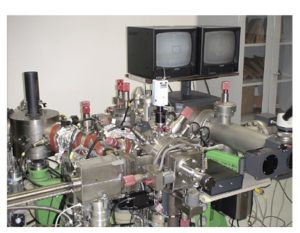## SIMS Analysis Tips – What is the effect of mass interference?

Secondary ion mass spectra measured by SIMS may not always provide information from the target element alone. If ions close to the mass of the target element exist, information other than the target element may be included depending on the type of mass spectrometer and mass resolution setting. Elements with similar mass numbers that affect the target element are called “interfering elements”.

## Quality nuisance element & mass decomposition energy

The factors that cause interference elements in SIMS analysis are the types of ion sources used for sputtering (oxygen / Cs), matrix (base material) elements, impurity elements contained in the matrix, atmospheric component elements in the SIMS system and the influence of multiply-charged ions can be considered.As an example, when measuring P in a Si substrate, which is used as a dopant, P with a mass number of 31 corresponds to Si with a mass number of 30, which is an isotope of Si, and H with a mass number of 1, which is a residual gas component in the apparatus. Because the mass number of the molecular ion formed by Si+H is 31, which is the same as P, the P measurement is affected by mass interference. Similarly, when analyzing As with a mass number of 75, which is a dopant in a Si substrate, the molecular ion mass of 75 (16O29Si30Si) is an interfering element.

## Examples of interfering elements (in Si materials)

Analytical ionInterfering ionMass resolution required for separation (M / △ M)
27AI +(12C2H3)+670
31P-(30SiH) –3950
32S+(16O2)+1810
56Fe+(28Si2) +2950
58Ni +(28Si30Si) +3760
63Cu +(28Si35Cl)-3890
As-(29Si30Si16O) –3180
As-(47Ti28Si) –10940

In order to perform measurements that are not affected by interfering elements, the “mass resolution” of the mass spectrometer must be set to a resolution that allows the target element and interfering elements to be separated. Mass resolution, the half-width of the mass spectrum is defined in “m / △ m” using the difference between two mass of interest. For example, when measuring Fe in Si, using a mass 56 Fe (56Fe: 55.93490) with a large isotope abundance ratio, 28Si2 (28Si + 28Si: 55.95386) with the same mass 56 becomes an interfering element. Configuring mass resolution in order to separate both (m / △ m) is 2950 or more (56 / 0.01896 or higher) is required.

The SIMS mass spectrometer used for depth analysis is a double-focusing mass spectrometer (magnetic SIMS) or a quadrupole mass spectrometer (quadrupole SIMS). Please refer to this link for the device configuration. Mass resolution of the magnetic type SIMS is “300-10000”, and approximately “300-1000” in quadrupole SIMS. We, therefore use magnetic sector SIMS capable of obtaining high mass resolution when analyzing an element which is subject to a mass interference.

In the case that contains the unknown material and the high concentration of impurities, it may be difficult to predict a mass interference in advance. The example, when data are greatly different from expectations, it is always wise to consider the possibility of a mass interference.